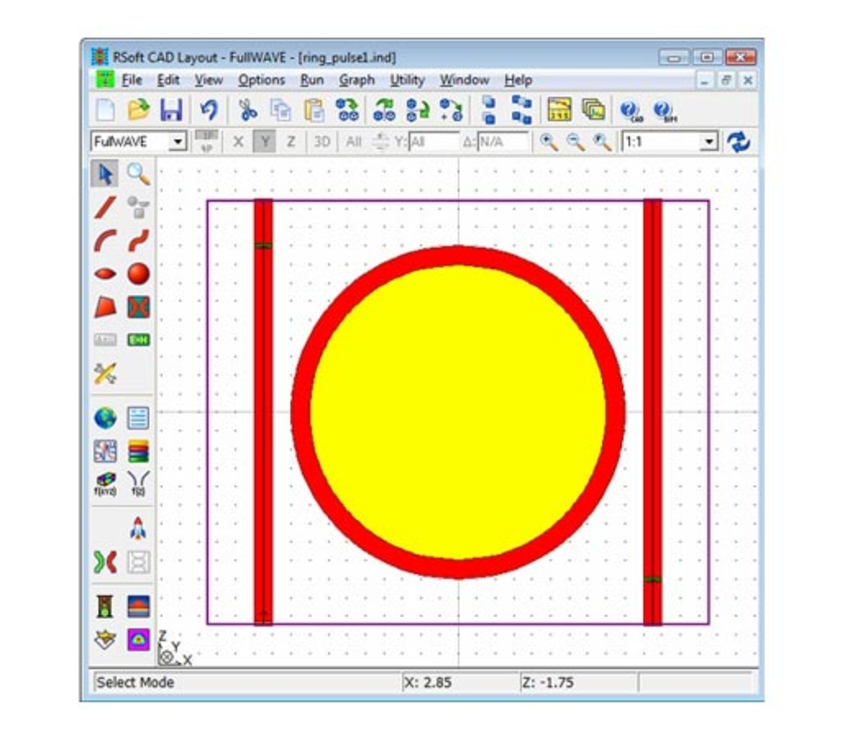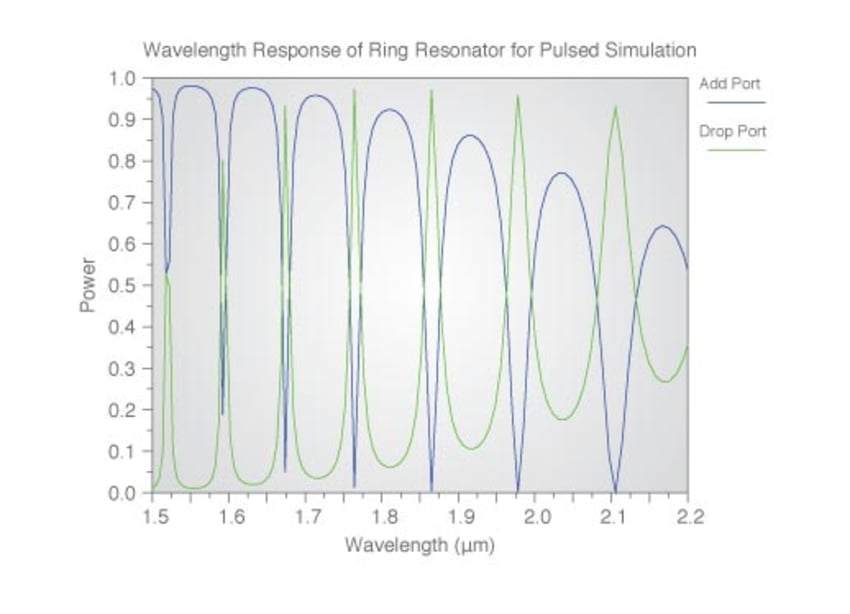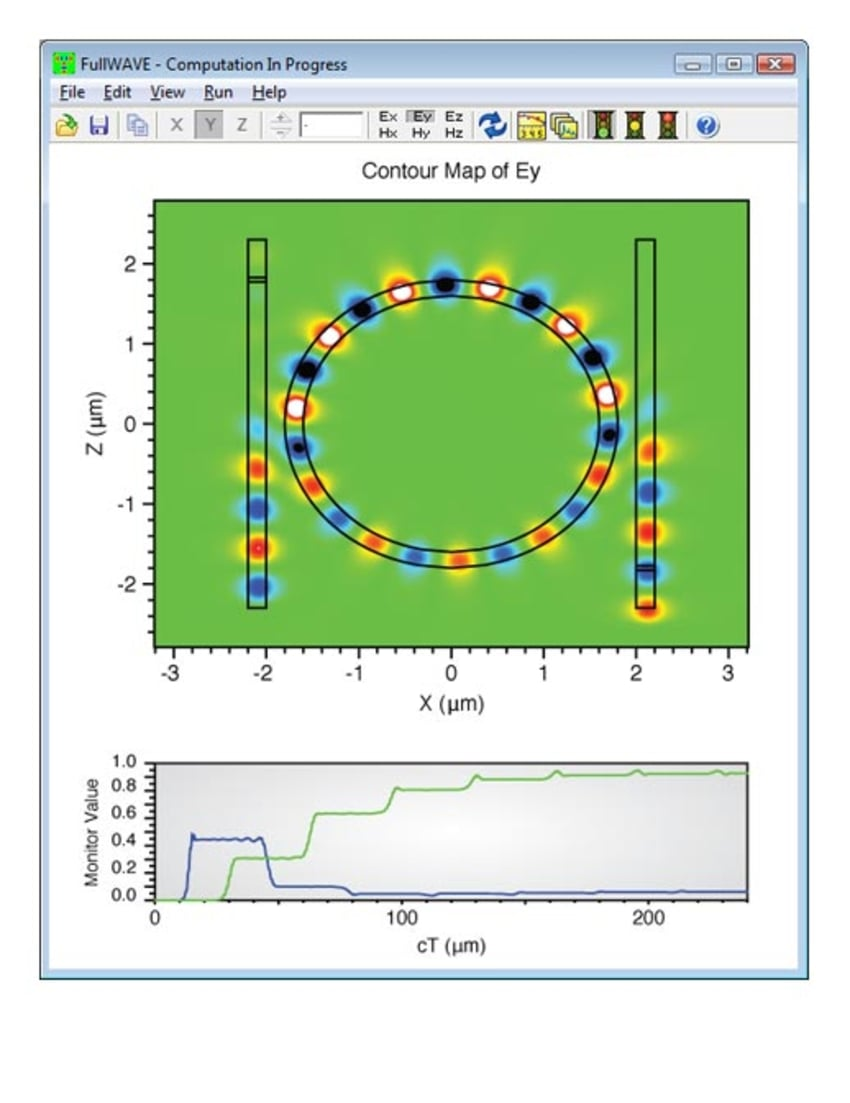# RSoft Application Gallery Note: Modeling a Ring Resonator

## Tool Used: FullWAVE

A ring resonator is a very useful device that acts as a high-Q filter for applications that require wavelength selectivity. This type of device has many resonances, and only these resonant wavelengths are coupled to the output waveguide. All other wavelengths are allowed to pass uncoupled through the input waveguide, hence the ring resonator is essentially an optical notch filer, with numerous stop bands.

In this application example, a ring resonator will be analyzed using FDTD. First, a pulse response is used to determine the spectrum of resonances, and then a CW simulation is used to demonstrate the propagation through the device, at one of the resonant wavelengths.

## Device Layout

The structure used in this example consists of waveguides that are 0.2 μm in width, and have a refractive index of 3. We will be looking in detail at resonances around a wavelength of 2 μm. These waveguides are separated from the ring by 0.2 μm, and the inner and outer radii of the ring are R-width/2, and R2=R+width/2, respectively, where R=1.7 μm.

The structure appears in the RSoft CAD as:where the green objects are time monitors used for analysis. The input signal enters the lower left port and exits (except for the resonant wavelengths) from the upper left port. The resonant wavelengths exit from the lower right port.

## Simulation: Pulsed Excitation

We will first calculate a wavelength/frequency spectrum for the ring resonator by using a pulsed excitation. The launch field will be the fundamental mode of the input port with a temporal FWHM equal to the wavelength in μm. When the simulation is complete, a Fourier transform is performed on the data stored by the time monitors to produce the wavelength response of the device.In the above figure, the green line represents the through port, and the blue line represents the drop port.

## Simulation: CW Excitation

We will now focus on the resonance around 1.977 μm shown in the wavelength response. To do this, we are going to set the wavelength to this value and use a CW simulation. The simulation results for this are:where the lower portion of the simulation window shows the time monitor results (in the time domain). At the end of the simulation, in steady-state, these results show that the energy in the ring has built up and almost the entire field is propagating in the output waveguide. By utilizing both the pulsed and CW calculations, we have calculated the spectral response of this device and illustrated the CW operation of the device at a resonance.